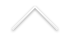When studying objects of a certain kind in any discipline, mathematical or not, one of the most relevant tasks is the classification of those objects. In topology the typical objects under study are spaces of various kinds, continuous maps, differentiable maps etc, and algebraic topology deals with the various classification problems via algebraic methods. One associates an algebraic invariant, such as a number or a group, to a topological object in such a way that when two objects are "equivalent" then the associated algebraic object is the same. As an example, closed orientable connected surfaces are classified by the genus. The ideas behind those methods have deep interactions with several areas of mathematics, such as symplectic geometry, differential geometry, dynamical systems and algebraic geometry.

The research team at UFRJ works on homological methods in symplectic geometry that arise from Morse theory for certain infinite dimensional functionals. The critical points of these functionals are periodic orbits of Hamiltonian systems and the study of the corresponding homologies has provided a new and powerful insight in Hamiltonian dynamics.

The main areas of research at UFRJ are currently the following:

• Contact homology and dynamics of Reeb flows.
• Pseudo-holomorphic curves and open-book decompositions.
• Floer homology for Lagrangian intersections.
• Symplectic capacities and periodic orbits on prescribed energy levels.
• Morse theory for the Lagrangian action functional.